an introduction to Principal Component Analysis PCA abstract

• Slides: 12an introduction to Principal Component Analysis (PCA)abstract Principal component analysis (PCA) is a technique that is useful for the compression and classification of data. The purpose is to reduce the dimensionality of a data set (sample) by finding a new set of variables, smaller than the original set of variables, that nonetheless retains most of the sample's information. By information we mean the variation present in the sample, given by the correlations between the original variables. The new variables, called principal components (PCs), are uncorrelated, and are ordered by the fraction of the total information each retains.Geometric picture of principal components (PCs) A sample of n observations in the 2 -D space Goal: to account for the variation in a sample in as few variables as possible, to some accuracyGeometric picture of principal components (PCs) • the 1 st PC is a minimum distance fit to a line in • the 2 nd PC is a minimum distance fit to a line in the plane perpendicular to the 1 st PC space PCs are a series of linear least squares fits to a sample, each orthogonal to all the previous.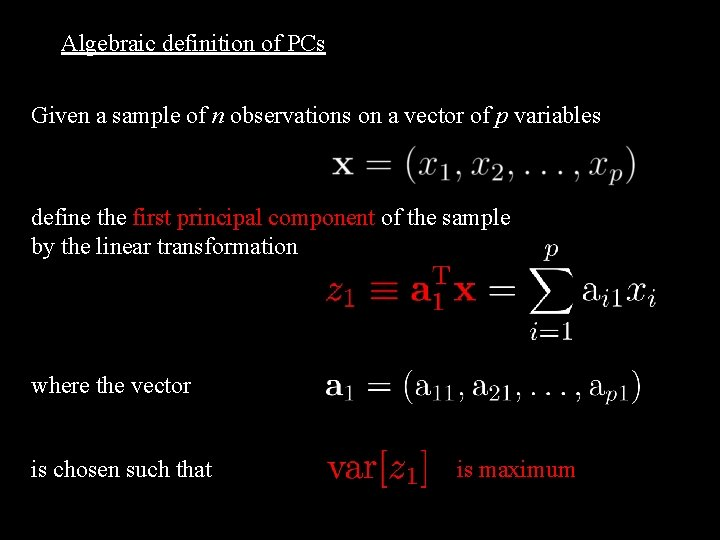Algebraic definition of PCs Given a sample of n observations on a vector of p variables define the first principal component of the sample λ by the linear transformation where the vector is chosen such that is maximum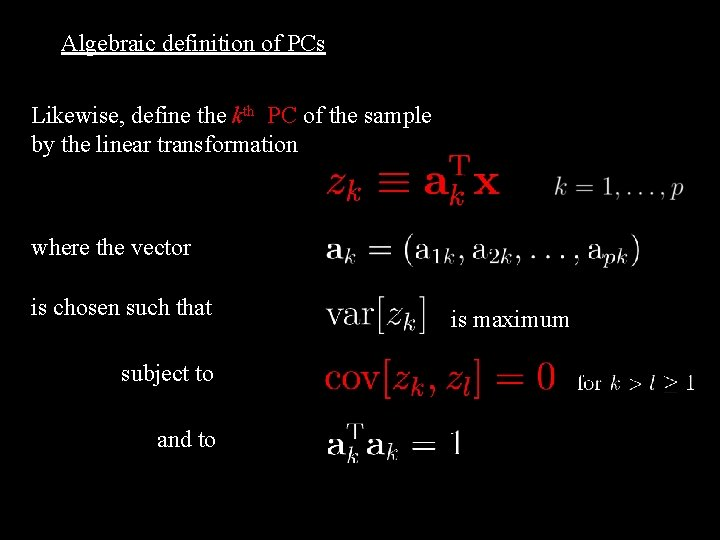Algebraic definition of PCs Likewise, define the kth PC of the sample by the linear transformation where the vector is chosen such that subject to and to λ is maximum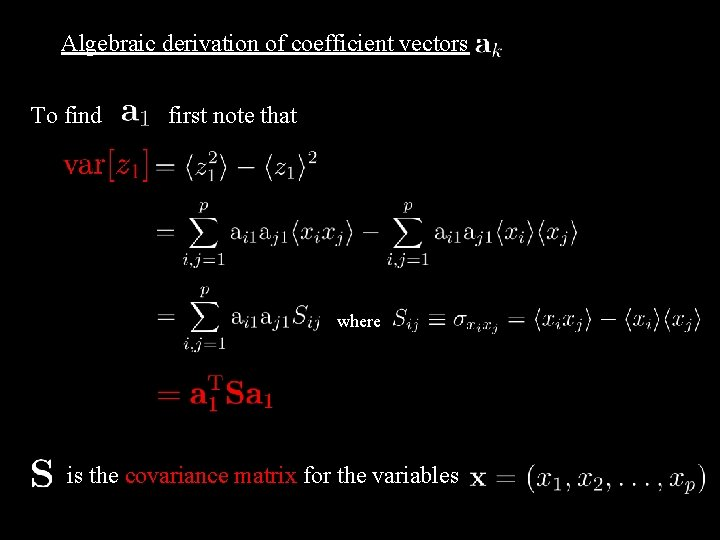Algebraic derivation of coefficient vectors To find first note that where is the covariance matrix for the variables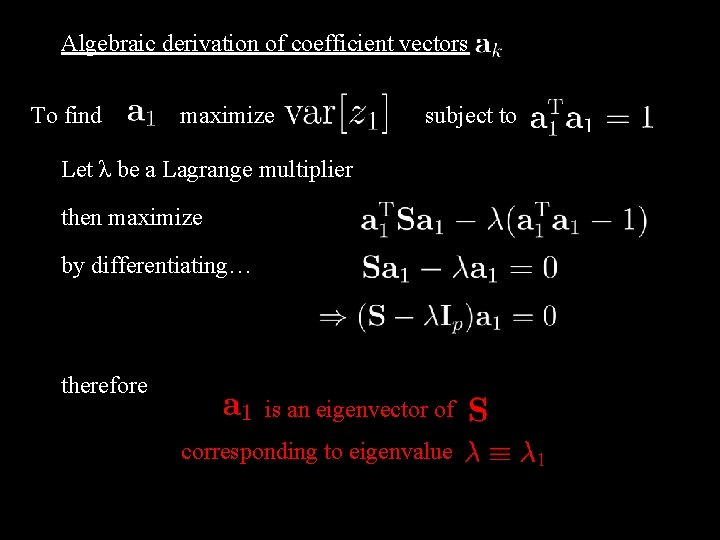Algebraic derivation of coefficient vectors To find maximize subject to Let λ be a Lagrange multiplier then maximize by differentiating… therefore is an eigenvector of corresponding to eigenvalue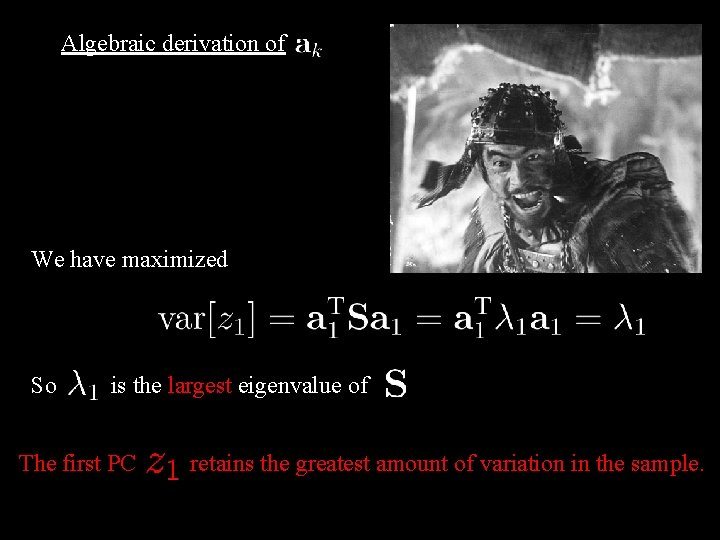Algebraic derivation of We have maximized So is the largest eigenvalue of The first PC retains the greatest amount of variation in the sample.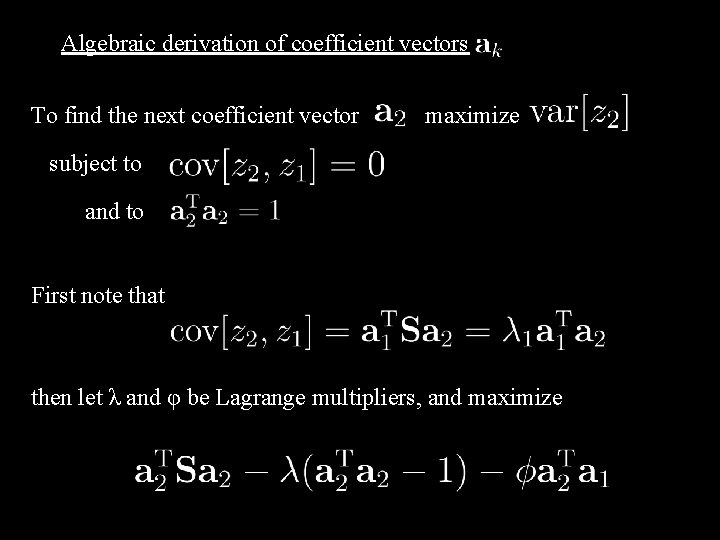Algebraic derivation of coefficient vectors To find the next coefficient vector maximize subject to and to First note that then let λ and φ be Lagrange multipliers, and maximize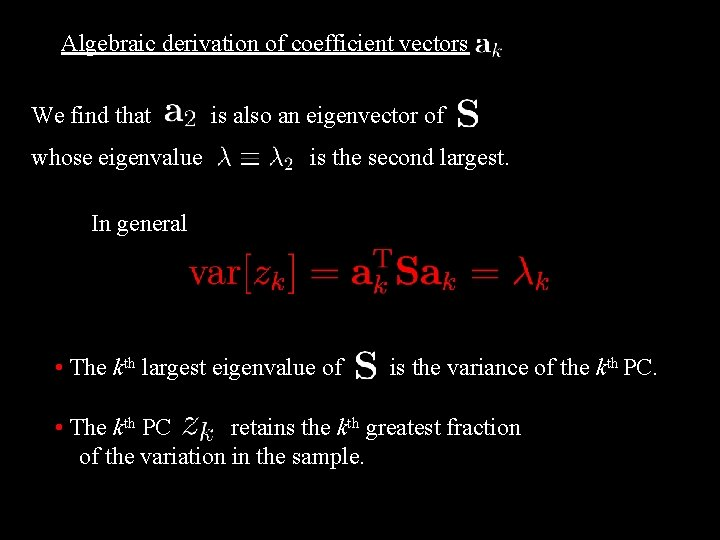Algebraic derivation of coefficient vectors We find that whose eigenvalue is also an eigenvector of is the second largest. In general • The kth largest eigenvalue of is the variance of the kth PC. • The kth PC retains the kth greatest fraction of the variation in the sample.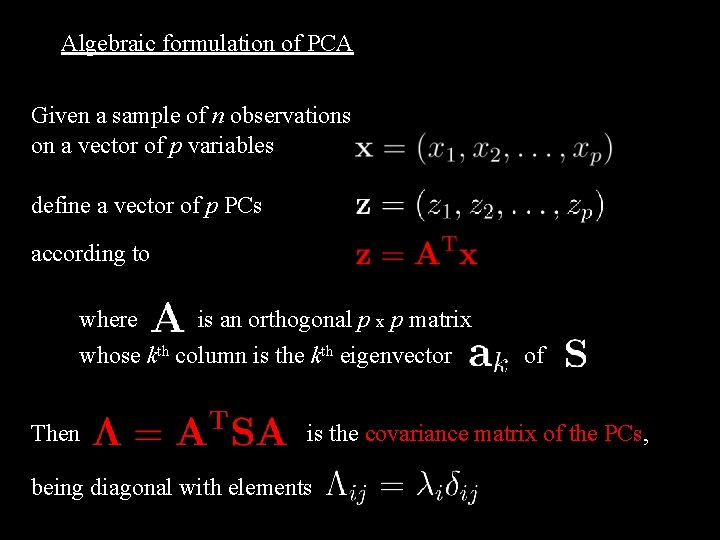Algebraic formulation of PCA Given a sample of n observations on a vector of p variables define a vector of p PCs according to where is an orthogonal p x p matrix whose kth column is the kth eigenvector Then of is the covariance matrix of the PCs, being diagonal with elements